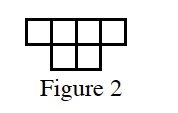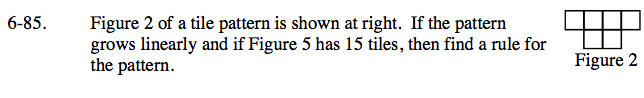### Home > CAAC > Chapter 6 > Lesson 6.2.5 > Problem6-85

6-85.

Figure 2 of a tile pattern is shown below. If the pattern grows linearly and if Figure 5 has 15 tiles, then find a rule for the pattern. 6-85 HW eTool (CPM). Homework Help ✎Make an x, y table with the x values representing the figure number and the y values representing the number of tiles.

Fill in values for x and y. What is the growth?

Work backward. What is the starting point?

Write an equation y = mx + b using the growth and starting point.

y = 3x

Does this rule work for your table?

Once you find a rule for the pattern use the eTool below to complete the figures.
Click the link at right for the full version of the eTool: AC 6-85 HW eTool.